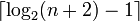# Panferov Lie group

## Definition

### For a ring and a natural number less than or equal to its powering threshold

Suppose$R$ is a commutative unital ring and$n$ is a natural number greater than or equal to 2 such that the additive group of$R$ is powered over the set of all natural numbers strictly less than$n$, or equivalently,$n$ is at most one more than the powering threshold of the additive group of$R$.

The Panferov Lie group for$R$ and$n$ is defined as the Lazard Lie group (via the Lazard correspondence) for the Panferov Lie algebra (defined below) with parameters$R$ and$n$.

### For a prime number: the extreme case

Let$p$ be a prime number. The Panferov Lie group for$p$ is the Panferov Lie group corresponding to$R = \mathbb{F}_p$ (the prime field) and$n = p$.

### Definition of Panferov Lie algebra being used

Suppose$n$ is a natural number greater than or equal to 2 and$R$ is a commutative unital ring. The Panferov Lie algebra of degree$n$ over the ring$R$ is a$R$-Lie algebra (and hence also is a Lie ring) defined as follows:

• The additive group is a free module of rank$n$ with basis$e_1,e_2,\dots,e_n$. Explicitly, the Lie algebra is$\bigoplus_{i=1}^n Re_i$
• The Lie bracket is defined as follows:$[e_i,e_j] = \left\lbrace \begin{array}{rl} (i - j)e_{i+j}, & i + j \le n \\ 0, & i + j > n \\\end{array}\right.$

## Particular cases

### Powering thresholds for some ring choices

Choice of$R$ Maximum permissible value of$n$ = one more than the powering threshold of$R$ (this is the smallest prime number such that$R$ is not powered over that number)$R = \mathbb{F}_p$, the prime field for a prime number$p$$p$$R = \mathbb{Q}$, the field of rational numbers no maximum, i.e.,$n$ could be any natural number.$R = \mathbb{F}_q$ where$q$ is a prime power that equals the size of the finite field$\mathbb{F}_q$ and$p$ is the underlying prime (the field characteristic).$p$$R = \mathbb{Z}/p^k\mathbb{Z}$ where$p$ is a prime number and$k$ is a positive integer.$p$

### Panferov Lie groups for some small choices of$n$$n$ Description of the Panferov Lie group with parameter$n$ Nilpotency class (equals$n - 1$) Derived length (equals$\lceil \log_2(n + 2) - 1 \rceil$) Theoretical maximum value of derived length for the nilpotency class (the theoretical maximum is$\lfloor \log_2(n - 1) + 1 \rfloor$) Is the derived length at the theoretical maximum for the nilpotency class? (No except for powers of 2 and Mersenne numbers, but otherwise off by one
2 additive group of$R \times R$. It is an abelian group. 1 1 1 Yes
3 unitriangular matrix group of degree three$UT(3,R)$ over$R$. 2 2 2 Yes
4 Panferov Lie group of degree four 3 2 2 Yes
5 Panferov Lie group of degree five 4 2 3 No
6 Panferov Lie group of degree six 5 2 3 No
7 Panferov Lie group of degree seven 6 3 3 Yes

### The extreme case

Prime number$p$ Panferov Lie group for$p$ Order (equals$p^p$) Nilpotency class (equals$p - 1$) Derived length (equals$\lceil \log_2(p + 2) - 1 \rceil$) Theoretical maximum value of derived length for the nilpotency class (the theoretical maximum is$\lfloor \log_2(p - 1) + 1 \rfloor$) Is the derived length at the theoretical maximum for the nilpotency class? (No except for$p = 2$ and Mersenne primes, in general it'll be off by one but still close enough)
2 Klein four-group 4 1 1 1 Yes
3 unitriangular matrix group:UT(3,3) 27 2 2 2 Yes
5 Panferov Lie group for 5 3125 4 2 3 No
7 Panferov Lie group for 7 823543 6 3 3 Yes

## Arithmetic functions

Function Value in the general case Value in the case$R = \mathbb{F}_p, n = p$ Explanation
order$|R|^n$$p^p$ same as the order of its Lazard Lie ring, which is$p$-dimensional vector space over$\mathbb{F}_p$.
exponent characteristic of the ring$R$$p$ same as the exponent of its Lazard Lie ring, which is$p$ on account of it being a nonzero vector space over$\mathbb{F}_p$.
nilpotency class$n - 1$$p - 1$ same as the nilpotency class of its Lazard Lie ring; see Panferov Lie algebra#Arithmetic functions
derived length$\lceil \log_2(n + 2) - 1 \rceil$$\lceil \log_2(p + 2) - 1 \rceil$ same as the derived length of its Lazard Lie ring, see Panferov Lie algebra#Arithmetic functions